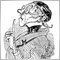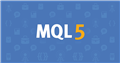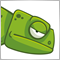# OOP issue : idea to break a vicious circle ?40779

Maybe it was already discussed, but I can't remember or find it.

Here is the problem :

EDIT: I am using int and double as examples in the code, but it had really to manage all basic types (and even complex ones actually), so including string for example.

1°I want to manage some variables of basic types (int, double, bool...) in some generic way to avoid repeating code, so I am using a template, and as we don't have pointers to basic types in mql, a wrapper class :

```template<typename T>
class CType
{
T                 value;
public:
CType(T v) : value(v) {};
~CType(void) {};
};
```
2° I want to manage these values in a collection or arrays, vector . . .so I need a parent class :
```class CParentType
{
//...
};

template<typename T>
class CType : public CParentType
{
//...
};
```

So I can use code like :

```   CParentType *values;
values=new CType<int>(INT_MIN);
values=new CType<double>(M_PI);
//...```

Ok, so far, so good.

Now, first problem I had (but I found a solution), is to initialize this array (or vector, whatever) dynamically. For example, I have a data file with values :

INT;157
DOUBLE;3.1415
...

I don't think there is an easy "standard" way, so I used an enum and a factory method.

```enum ENUM_TYPE { T_INT,T_DOUBLE,T_BOOL };
//---
CParentType*Factory(ENUM_TYPE t)
{
CParentType*vobj=NULL;
switch(t)
{
case T_INT    : vobj=new CType<int>(NULL); break;
case T_DOUBLE : vobj=new CType<double>(NULL); break;
//...
default:
break;
}
return(vobj);
}```

This is simplified code of course, I don't manage the initial value, just the type.

And I can initialize my array dynamically :

```   int X=10;
//--- basic data read in a file for example
ENUM_TYPE   types[];
CParentType *values[];
//--- dynamic initialization
for(int i=0;i<X;i++)
{
values[i]=Factory(types[i]);
}```

Still no real problem, but a nicer built-in solution in mql would be (but doesn't compile of course) :

```//--- would be nice mql built-in solution
for(int i=0;i<X;i++)
{
values[i]=new CType<types[i]>(NULL);
}```

So first question : is there a better solution than a factory method and an ENUM ?

Nota: I could have use the built-in mql enum ENUM_DATATYPE instead of a custom one, but that's a detail which doesn't change the problem).

Now, the vicious circle. I want to extract the original value as an mql basic type :

I added a Get method :

```   T CType::Get(void)   { return(value); };
```

But to call it I need to dynamic_cast the pointer stored.

```   CType<int>*variable=dynamic_cast<CType<int>*>(values);
int originalValue    = variable.Get();```

Problem is 'int' is not known (from a dynamic point of view)

The actual basic type when you have a CParentType pointer like values[i] is unknown, so once again, I would need to use ENUM_TYPE and a switch or try one by one all possible dynamic cast, but I would need to repeat it for each type.

Example, imagine I have to call a functionA with an int parameter, or a functionB with a double parameter.

```void functionA(int value)     { /*...*/  }
void functionB(double value)  { /*...*/  }```

I will need to declare a variable for any type, then call the "dynamic cast" function, trying one by one :

```//--- need to call functionA or functionB
int originalIntValue       = NULL;
double originalDoubleValue = NULL;

if(ViciousCircle(values,originalIntValue))
{
functionA(originalIntValue);
}
//--- Ok but what if know I want to call functionB if the original value is double ?
else if(ViciousCircle(values,originalDoubleValue))
{
functionB(originalDoubleValue);
}
// Imagine that for each basic type and for any functionX !!!```

So the starting generic approach leads to further need to repeat code again and again.

Maybe I am too focused on my project and there is an other solution, but I missed it for now. Any idea ?1200

In Basic there's the Variant type. You could emulate it like this:

```union VariantValue {
bool as_bool;
int  as_int;
long as_long;
double as_double;
};

class Variant {
ENUM_TYPE type;
VariantValue value;
public:
// constructors
Variant(bool bval)   { type=T_BOOL; value.as_bool=bval; };
Variant(int ival)    { type=T_INT; value.as_int=ival; };
Variant(long lval)   { type=T_LONG; value.as_long=lval; };
Variant(double dval) { type=T_DOUBLE; value.as_double=dval; };

// getters
bool   Variant::GetBool()   { return value.as_bool; };
int    Variant::GetInt()    { return value.as_int; };
long   Variant::GetLong()   { return value.as_long; };
double Variant::GetDouble() { return value.as_double; };
};```

I don't know if it compiles right away, but in principle this should work. You could also build arrays/lists/hashes from it.40779

I don't think there is a solution keeping the original generic approach (templates and parent class), as I can't apply polymorphism on template. I probably need to remove the template at the beginning.40779

lippmaje:

In Basic there's the Variant type. You could emulate it like this:

I don't know if it compiles right away, but in principle this should work. You could also build arrays/lists/hashes from it.

Thanks for your answer. Unfortunately it's just an other way to have similar issue(s) as you need to know the type to call the right method.

I need a generic AND dynamic approach, and mql is missing some features to do it easily. However I am still hoping to find a nice solution without them.Moderator
10536

1°I want to manage some variables of basic types (int, double, bool...) in some generic way to avoid repeating code, so I am using a template, and as we don't have pointers to basic types in mql, a wrapper class :

I guess that if this were possible in MQ5 MQ would have used that for its own functions! But e.g.  fmax() from here exists for each basic type.

As one can see for each type its own overloaded function exists.

What might do it is casting all the variable into the same basic type - if the numbers are all small enough and you don't get trouble with negative numbers ...• www.mql5.com
Usually the function name tends to reflect its main purpose. As a rule, readable programs contain various well selected identifiers. Sometimes different functions are used for the same purposes. Let's consider, for example, a function that calculates the average value of an array of double precision numbers and the same function, but operating...46202

You can solve the "New" issue with a template method.

```template<typename T> CType<T> NewCType(T val)
{
return new CType<T>(val);
}```

If you must use a base class then you should add a virtual method that gets a value for all the derived classes (in this case it should return a double)

`virtual double Get();`

However this isn't really the best option for storing different sizes of numbers in a vector

I'd just use an array of doubles because using polymporphism to store different sizes of built in types isn't really what it was invented for1200

Alain Verleyen:

Thanks for your answer. Unfortunately it's just an other way to have similar issue(s) as you need to know the type to call the right method.

I need a generic AND dynamic approach, and mql is missing some features to do it easily. However I am still hoping to find a nice solution without them.

I think what you are referring to is called Boxing/Unboxing in OOP.

```void Variant::Get(bool &bval)   { bval=value.as_bool; }
void Variant::Get(int &ival)    { ival=value.as_int; }
void Variant::Get(long &lval)   { lval=value.as_long; }
void Variant::Get(double &dval) { dval=value.as_double; }
```

This is simple function overloading like with the constructors. While boxed you can pass them around in their Variant container as long as you need to. But in the end, when it comes to address the values, you always have to be precise about the base type, that's called unboxing.40779

Carl Schreiber:

I guess that if this were possible in MQ5 MQ would have used that for its own functions! But e.g.  fmax() from here exists for each basic type.

As one can see for each type its own overloaded function exists.

What might do it is casting all the variable into the same basic type - if the numbers are all small enough and you don't get trouble with negative numbers ...

I need a solution for the whole of my requirements. Point 1° is only a little part and of course I simplified here to stay relatively clear and simple.

Function overloading is not applicable of or no help in my case.40779

James Cater:

You can solve the "New" issue with a template method.

What "new" issue ? You mean the usage of a Factory method with an enum ?

If it's what you mean I can't as it has to be dynamic, my data comes from a TXT file, they are strings and I know the real type from the value provided in the file.

If you must use a base class then you should add a virtual method that gets a value for all the derived classes (in this case it should return a double)

However this isn't really the best option for storing different sizes of numbers in a vector

I'd just use an array of doubles because using polymporphism to store different sizes of built in types isn't really what it was invented for

I realize that my description was incomplete or misleading. I used int/double as example, but it could be any data type including string and actually the parent class could even be derived with non-basic types. So for example you could have :

`class CComplexType : public CParentType`
In conclusion I can't use an array of doubles, as it really needs to manage generic data types.40779

lippmaje:

I think what you are referring to is called Boxing/Unboxing in OOP.

This is simple function overloading like with the constructors. While boxed you can pass them around in their Variant container as long as you need to. But in the end, when it comes to address the values, you always have to be precise about the base type, that's called unboxing.

See my answer to James. Unfortunately I mislead you by using only int and double in the example, but it has to manage all basic types (and more), sorry.

So an 'union' cannot be used, however your "variant" approach could be interesting but would need a "custom union", I will think about it. Thanks.1200

How about processing the generic value with a function array.

```void handleBool  (Variant var) { Print(var.GetBool()); }
void handleInt   (Variant var) { Print(var.GetInt()); }
void handleString(Variant var) { Print(var.GetString()); }

typedef void (*TVariantHandler)(Variant);
TVariantHandler handlers[MAX_TYPES];

void init() {
handlers[T_BOOL]=handleBool;
handlers[T_INT]=handleInt;
handlers[T_STRING]=handleString;
}

void process(Variant var) {
TVariantHandler handler=handlers[var.type];
handler(var);
}```
And yes, you could build a 'custom' union to hold more complex types.